GeeksforGeeks App
Open AppBrowser
Continue

# Methods of charging Depreciation

Depreciation refers to the decrease in the value of assets of the company over the time period due to use, wear and tear, and obsolescence. In others words, It is the method to allocate the cost of an asset over its useful life. Depreciation is always charged on the cost price of the asset and not on its market price. It is charged every year to the extent of the depreciable amount. Examples of assets that can be depreciated are Machines, Computers, Furniture, Vehicles, etc.

According to R.N. Carter, “Depreciation is the gradual and permanent decrease in the value of an asset from any cause.”

According to William Pickles, “Depreciation may be defined as the permanent and continuing diminution in the quality, quantity or the value of an asset.”

### Methods of Calculating Depreciation:

Businesses choose different methods for calculating depreciation according to their need. The two most prominent methods for calculating depreciation are the Straight Line Method and the Diminishing Balance Method.

### 1. Straight Line Method:

Under this method of charging depreciation, the amount charged as depreciation for any asset is fixed and equal for every year. The amount of depreciation is deducted from the original cost of an asset and charged on the debit side of the Profit and Loss A/c as a loss. The concerned asset is depreciated with an equal amount every year until the book value of the asset becomes equal to the scrap value of the asset. It is also called the ‘Equal Installment Method’ or ‘Fixed Installment Method’.

#### Merits of Straight Line Method:

1. Simplicity: The calculation of depreciation under this method is not much complicated. The simplicity of the method is also a reason behind this method being widely popular.

2. Equality of Depreciation Burden: In this method, the depreciation charged for every year is equal. Hence, the amount transferred to Profit and Loss A/c for each year is equal, and the burden of depreciation on the Net Profit of the company for every year will be the same.

3. Assets can be Completely Written off: The book value of the asset under this method can be depreciated until it becomes zero or equal to the scrap value of the asset. This helps companies in writing off an asset completely.

4. Knowledge of Original Cost and Up-to-date Depreciation: Under this method, the original cost of the asset is shown in the balance sheet along with the deduction of the depreciation for the year. This helps businesses in keeping up with the information regarding depreciation for the year and the actual cost.

#### Formula for Calculating Depreciation:

1. When Scrap Value is Given:2. When Rate of Depreciation is Given:#### Journal Entries:

1. At the time of Purchase of Fixed Assets: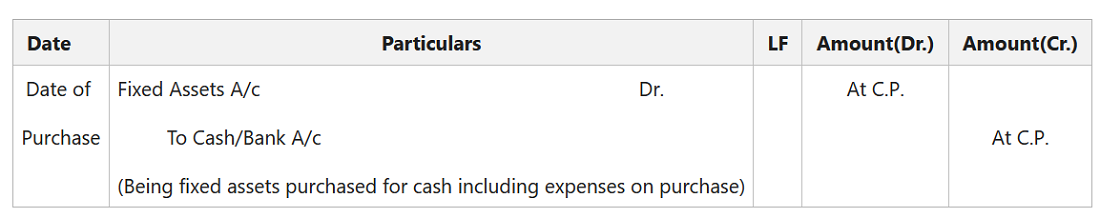2. At the End of Each Accounting Period:

(i) For the Depreciation charged on Fixed Assets: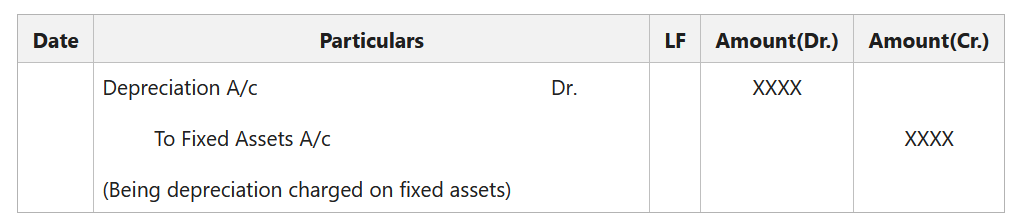(ii) For Transferring Amount of Depreciation to Profit & Loss A/c: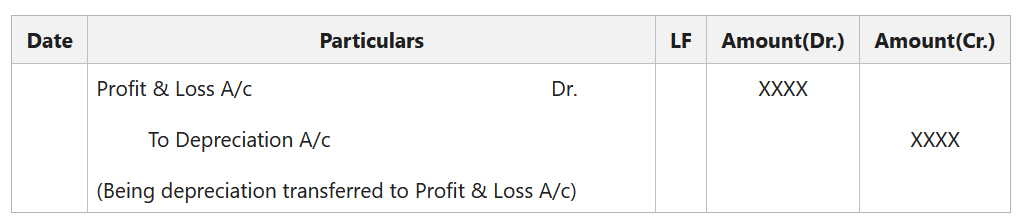3. At the Time of Sale of Fixed Assets:

(i) For the Depreciation charged on Selling Assets (Up to the Date of Sale):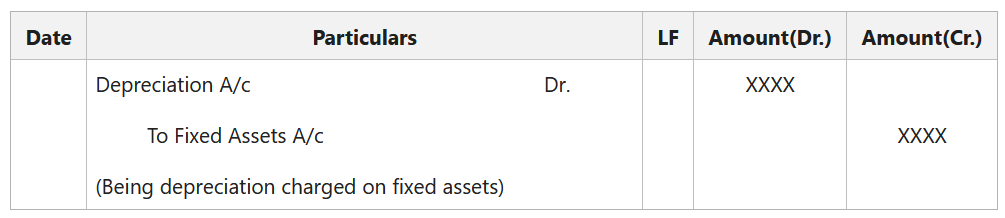(ii) For the Sale of Fixed Assets: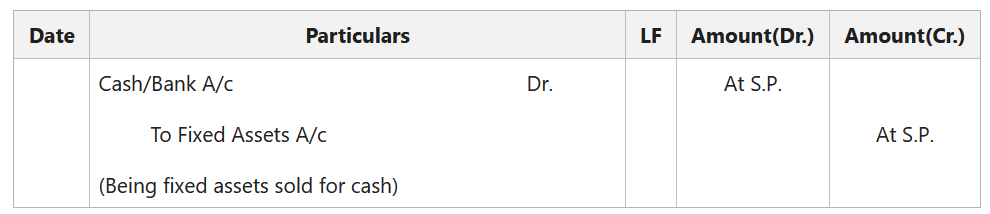(iii) For the Profit/Loss on Sale of Fixed Assets:

• For the Profit on Sale of Fixed Assets (If Sale Price is More than the Book Value):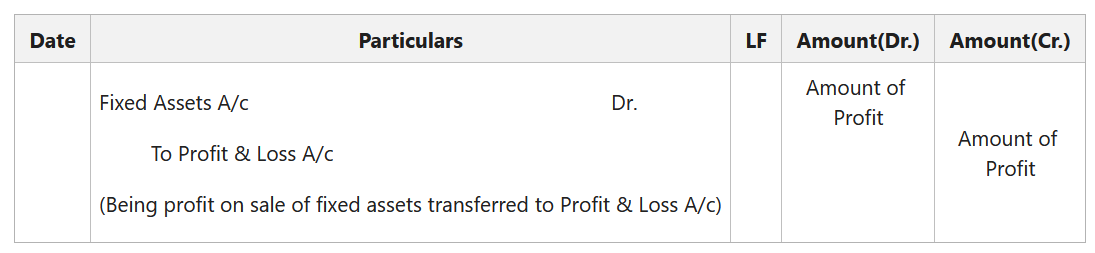• For the Loss on Sale of Fixed Assets (If Sale Price is Less than the Book Value):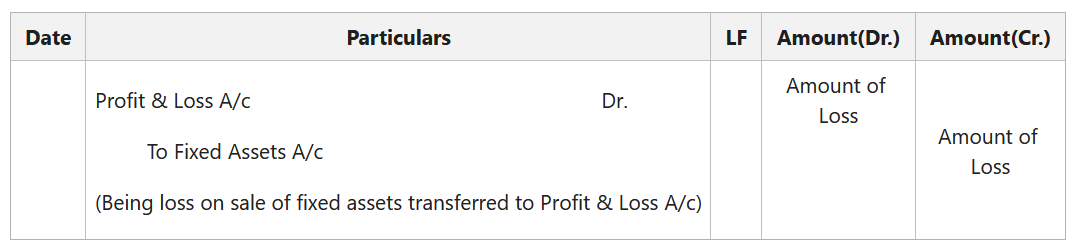### 2. Written Down Value Method:

Under this method of charging depreciation, the amount charged as depreciation for any asset is charged at a fixed rate, but on the reducing value of the asset every year. The amount of depreciation is deducted from the written down value (i.e., cost less depreciation) of an asset and charged on the debit side of the Profit and Loss A/c as a loss. The concerned asset is depreciated with an unequal amount every year, as the depreciation is charged to the book value and not to the cost of the asset.

#### Merits of Written Down Value Method:

1. Based on Logical Assumption: In the Written Down Value Method, the depreciation is charged more in earlier years of use of an asset, unlike the Straight Line Method in which depreciation is charged equally for every year. This is based on a logical assumption that assets will be used more in the initial years.

2. Suitability: This method is suitable for those assets that require high maintenance and repair cost and the later stage of their life.

3. Equal Charge Against Income: In the initial years of an asset, the depreciation charged under this method will be higher and repair charges due to wear and tear of the asset will be low. After some years, when the asset becomes older, the amount of depreciation charged will be less, but the amount spent on repair becomes high. Because of this, the burden on the business related to depreciation and repairs combined remains almost the same every year.

4. Recognised by Authorities: This method of charging depreciation is recognised by various government authorities, including Income Tax Department.

#### Formula for Calculating Depreciation:

1. When Scrap Value is Given (To find the rate of depreciation)2. When Rate of Depreciation is Given:#### Journal Entries:

1. At the time of Purchase of Fixed Assets:2. At the End of Each Accounting Period:

(i) For the Depreciation charged on Fixed Assets: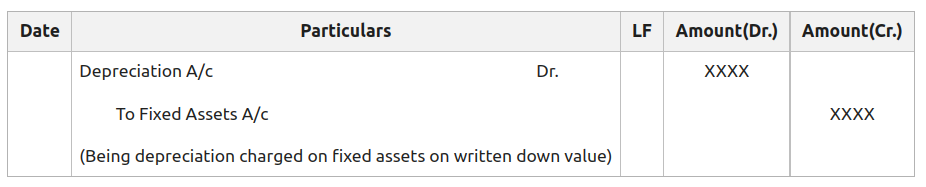(ii) For Transferring Amount of Depreciation to Profit & Loss A/c:3. At the Time of Sale of Fixed Assets:

(i) For the Depreciation charged on Selling Assets (Up to the Date of Sale):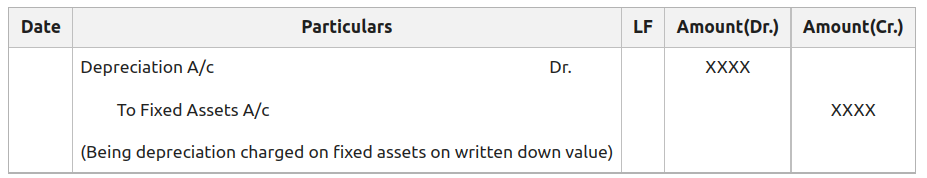(ii) For the Sale of Fixed Assets:(iii) For the Profit/Loss on Sale of Fixed Assets:

• For the Profit on Sale of Fixed Assets (If Sale Price is More than the Book Value):• For the Loss on Sale of Fixed Assets (If Sale Price is Less than the Book Value):My Personal Notes arrow_drop_up
Related Tutorials